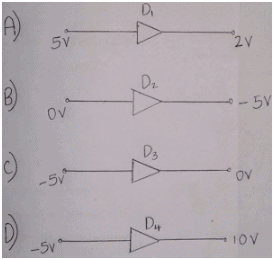Courses

# Test: Quantitative Theory Of The PN Diode Currents

## 10 Questions MCQ Test Electronic Devices | Test: Quantitative Theory Of The PN Diode Currents

Description
This mock test of Test: Quantitative Theory Of The PN Diode Currents for Electrical Engineering (EE) helps you for every Electrical Engineering (EE) entrance exam. This contains 10 Multiple Choice Questions for Electrical Engineering (EE) Test: Quantitative Theory Of The PN Diode Currents (mcq) to study with solutions a complete question bank. The solved questions answers in this Test: Quantitative Theory Of The PN Diode Currents quiz give you a good mix of easy questions and tough questions. Electrical Engineering (EE) students definitely take this Test: Quantitative Theory Of The PN Diode Currents exercise for a better result in the exam. You can find other Test: Quantitative Theory Of The PN Diode Currents extra questions, long questions & short questions for Electrical Engineering (EE) on EduRev as well by searching above.
QUESTION: 1

### What is the thickness of ‘space charge region’ or ‘transition region’ in P-N junction diode?

Solution:

The region of the junction is depleted by mobile charges, hence it is called space charge region or depletion region or transition region which is 10-4 cm = 10-6 m= 1 micron.

QUESTION: 2

### If what of the following is doped into a semiconductor say germanium a P-N junction is formed.

Solution:

A P-N junction is formed only when a donor impurities and acceptor impurities are added to either side of a semiconductor like silicon and germanium.

QUESTION: 3

### Which of the factors doesn’t change the diode current.

Solution:

I = Io [e(v/nVt) -1], as shown in this equation the diode current is dependent on temperature , voltage applied on the diode , Boltzmann’s constant but diode current is not dependent on resistance as it is independent of resistance.

QUESTION: 4

The product of mobility of the charge carriers and applied Electric field intensity is known as

Solution:

When the semiconductors like silicon and germanium is implied by an electric field the charge carriers when get drifted by certain velocity known as drift velocity. Drift velocity is product of mobility of charge carriers and field intensity.

QUESTION: 5

The tendency of charge carriers to move from a region of heavily concentrated charges to region of less concentrated charge is known as.

Solution:

In a semiconductor the charge will always have a tendency to move from higher concentrated area to less concentrated area to maintain equilibrium this movement of charges will result in diffusion current.

QUESTION: 6

If the drift current is 100mA and diffusion current is 1A what is the total current in the semiconductor diode.

Solution:

We know that the total current in a semiconductor is equal to sum of both drift current and diffusion current. Total current = 1A + 100mA =1.1A.

QUESTION: 7

Which of the following is reverse biased?Solution:

The P-N junction diode is forward bias when the voltage applied to p type is greater than the n type and vice versa, since the voltage applied to p type is less in C) it is the answer.

QUESTION: 8

The drift velocity is 5V and the applied electric field intensity 20v/m what will be the mobility of charge carriers.

Solution:

We know that mobility of charge carriers is drift velocity divide by applied electric field intensity. Mobility = drift velocity / field intensity.

QUESTION: 9

When there is an open circuit what will be the net hole current.

Solution:

If there is any current present under open circuit there will be an indefinite growth of holes at one end of the semiconductor which is practically not possible hence zero amperes.

QUESTION: 10

Rate of change of concentration per unit length in a semiconductor is called as.

Solution:

In a semiconductor the holes as well as electrons which are the charge carriers is not equally concentrated on all regions of the semiconductor the change in their rate is referred as concentration gradient.